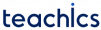# Tree Data Structure

A tree is a non-linear data structure that organizes data in a hierarchical structure and this is a recursive definition. It is a set of one or more nodes, with one node identified as the tree’s root and all remaining nodes partitionable into non-empty sets, each of which is a subtree of the root.

Since it is a non-linear data structure, different tree data structures provide for faster and easier access to the data.

## Tree Terminologies

#### Node

A node is a unit of data that contains a key or value as well as pointers to its child nodes.

#### Root

The topmost node in a tree is called the root node. This is where the tree is originated from.

#### Parent Node

A parent node or ancestor is a node that has a branch from it to another node.

#### Child Node

A child node (descendant or successor) is a node that is a descendent of another node.

#### Sibling Nodes

Nodes that have the same parent are called sibling nodes.

#### Leaf Node and Internal Node

The node which does not have any child is called a leaf node or terminal node. The nodes having at least a child node is called an internal node.

#### Level of a Tree

Each step from top to bottom in a tree is referred to as the level of a tree.

#### Degree

The total number of children of a node is the degree of a node. The number of edges arriving at that node is called the in-degree of a node. The number of edges leaving that node is the out-degree of a node.

The highest degree of a node among all the nodes in the tree is referred to as the degree of the tree.

#### Depth

The total number of edges from the root node to a particular node is called the depth of that node.

#### Height

The height of a node is the total number of edges that lie on the longest path from the node to any leaf node.

The height of a tree is the height of the root node.

## Types of Tree

• Binary Tree
• Binary Search Tree
• AVL Tree
• B-Tree
• Expression trees
• Tournament trees

## Applications of Tree

• To search an element in a set quickly, Binary Search Trees(BSTs) are used.
• Heap sort is done by a kind of tree called Heap.
• Tries are modified version of trees used to store routing informations in routers.
• Compiler use a syntax tree to parse program you write.
• Shortest path trees and spanning Trees are used in bridges and routers.
Subscribe
Notify of